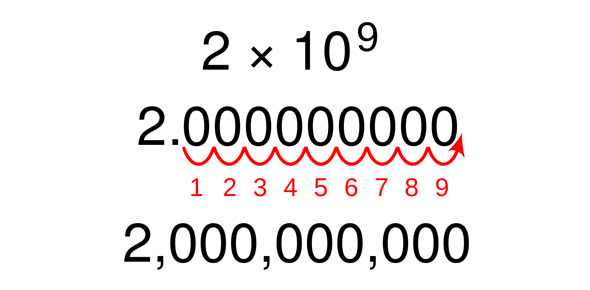# Expanded Notation 1

9 Questions | Total Attempts: 264Settings3rd grade quiz on expanded form of numbers to 10,000

Related Topics
• 1.
Which number is missing?9,000 + ____ + 4 = 9,804
• A.

8,000

• B.

800

• C.

80

• D.

8

• 2.
Which number is missing?__ + 500 + 60 + 8 = 4,568
• A.

40,000

• B.

4,000

• C.

400

• D.

40

• 3.
Which number is missing?2,000 + 500 + __ + 9 = 2,589
• A.

8,000

• B.

800

• C.

80

• D.

8

• 4.
Which number is missing?3,000 + __ = 3,070
• A.

7,000

• B.

700

• C.

70

• D.

7

• 5.
Type this number in standard form8,000 + 20 + 5
• 6.
Which number means2,000 + 900 + 8
• A.

298

• B.

2098

• C.

2908

• D.

2980

• 7.
Which of these equals 637?
• A.

6 + 3 + 7

• B.

6 + 30 + 7

• C.

600 + 30 + 7

• D.

60 + 30 + 7

• 8.
Which number equals 1,082 ?
• A.

1000 + 80 + 2

• B.

1 + 0 + 8 + 2

• C.

100 + 80 + 2

• D.

1000 + 800 + 2

• 9.
Type this number in standard form502